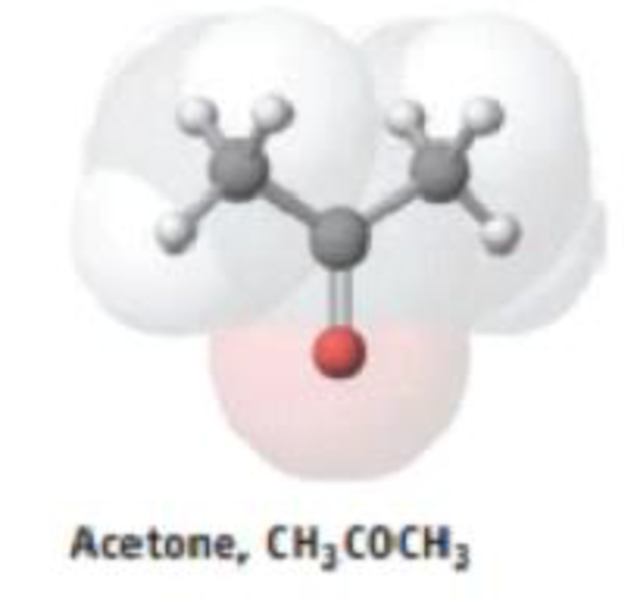Chapter 4, Problem 80GQ

Chapter
Section
Textbook Problem

The metabolic disorder diabetes causes a buildup of acetone, CH3COCH3, in the blood. Acetone, a volatile compound, is exhaled, giving the breath of untreated diabetics a distinctive odor. The acetone is produced by a breakdown of fats in a series of reactions. The equation for the last step, the breakdown of acetoacetic add to give acetone and CO2, isCH3COCH2CO2H →CH3COCH3 + CO2What mass of acetone can be produced from 125 mg of acetoacetic acid?

Interpretation Introduction

Interpretation:

The mass of acetone, CH3COCH3 produced from 125g of acetoacetic acid has to be determined.

Concept introduction:

• The relation between the number of moles and mass of the substance is ,

Numberofmole=MassingramMolarmass

Massingramofthesubstance=Numberofmole×Molarmass

• The molar mass of an element or compound is the mass in grams of 1 mole of that substance, and it is expressed in the unit of grams per mol (g/mol).
• For chemical reaction balanced chemical reaction equation written in accordance with the Law of conservation of mass.
• Law of conservation of mass states that for a reaction total mass of the reactant and product must be equal.
• Stoichiometric factor is a relationship between reactant and product which is obtained from the balanced chemical equation for a particular reaction.
Explanation

Balanced chemical equation for the given reaction is,

CH3COCH2CO2H CH3COCH3+ CO2

From the balanced chemical equation it is clear that acetoacetic acid, CH3COCH2CO2H and acetone, CH3COCH3 react in 1:1ratio.

The amount (moles) of acetoacetic acid, CH3COCH2CO2H   available in the reaction can be calculated as follows,

Amount of CH3COCH2CO2H available = 0.125gofCH3COCH2CO2H102.09g/molCH3COCH2CO2H =0.001molCH3COCH2CO2H

Using the stoichiometric factor the amount of acetone CH3COCH3 can be calculated

Still sussing out bartleby?

Check out a sample textbook solution.

See a sample solution

The Solution to Your Study Problems

Bartleby provides explanations to thousands of textbook problems written by our experts, many with advanced degrees!

Get Started

What is population genetics?

Human Heredity: Principles and Issues (MindTap Course List)

22-18 Draw the structures of L- and D-valine.

Introduction to General, Organic and Biochemistry

What is the maximum number of bonds that a carbon atom can form?

Biology: The Dynamic Science (MindTap Course List)